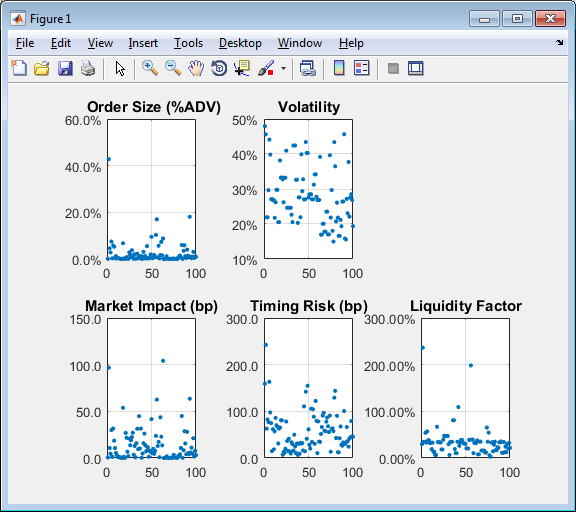Main Content

## Estimate Portfolio Liquidation Costs

This example shows how to determine the cost of liquidating individual stocks in a portfolio using transaction cost analysis from the Kissell Research Group. Compare the individual stocks in a portfolio using various metrics in a scatter plot.

The example data uses the percentage of volume trade strategy to calculate costs. You can also use the trade time trade strategy to run the analysis by replacing the percentage of volume data with trade time data.

To access the example code, enter `edit KRGPortfolioLiquidityExample.m` at the command line.

### Retrieve Market-Impact Parameters and Load Transaction Data

Retrieve the market-impact data from the Kissell Research Group FTP site. Connect to the FTP site using the `ftp` function with a user name and password. Navigate to the `MI_Parameters` folder and retrieve the market-impact data in the `MI_Encrypted_Parameters.csv` file. `miData` contains the encrypted market-impact date, code, and parameters.

```f = ftp('ftp.kissellresearch.com','username','pwd'); mget(f,'MI_Encrypted_Parameters.csv'); close(f) miData = readtable('MI_Encrypted_Parameters.csv','delimiter', ... ',','ReadRowNames',false,'ReadVariableNames',true);```

Create a Kissell Research Group transaction cost analysis object `k`.

`k = krg(miData);`

Load the example data `TradeData` from the file `KRGExampleData.mat`, which is included with the Datafeed Toolbox™.

`load KRGExampleData.mat TradeData`

For a description of the example data, see Kissell Research Group Data Sets.

### Estimate Trading Costs

Estimate market-impact costs `mi`.

`TradeData.mi = marketImpact(k,TradeData);`

Estimate the timing risk `tr`.

```TradeData.tr = timingRisk(k,TradeData); ```

Estimate the liquidity factor `lf`.

`TradeData.lf = liquidityFactor(k,TradeData);`

For details about the preceding calculations, contact the Kissell Research Group.

### Display Portfolio Plots

Create a scatter plot that shows the following:

• Size

• Volatility

• Market impact

• Timing risk

• Liquidity factor

```figure axOrder = subplot(2,3,1); nSymbols = 1:length(TradeData.Size); scatter(nSymbols,TradeData.Size*100,10,'filled') grid on box on title(' Order Size (%ADV)') axOrder.YAxis.TickLabelFormat = '%.1f%%'; axVolatility = subplot(2,3,2); scatter(nSymbols,TradeData.Volatility*100,10,'filled') grid on box on title('Volatility') axVolatility.YAxis.TickLabelFormat = '%g%%'; axMI = subplot(2,3,4); scatter(nSymbols,TradeData.mi,10,'filled') grid on box on title('Market Impact (bp)') axMI.YAxis.TickLabelFormat = '%.1f'; axTR = subplot(2,3,5); scatter(nSymbols,TradeData.tr,10,'filled') grid on box on title('Timing Risk (bp)') axTR.YAxis.TickLabelFormat = '%.1f'; axLF = subplot(2,3,6); scatter(nSymbols,TradeData.lf*100,10,'filled') grid on box on title('Liquidity Factor') axLF.YAxis.TickLabelFormat = '%.2f%%';```This figure demonstrates a snapshot view into the trading and liquidation costs, volatility, and size of the stocks in the portfolio. You can modify this scatter plot to include other variables from `TradeData`.

Download ebook